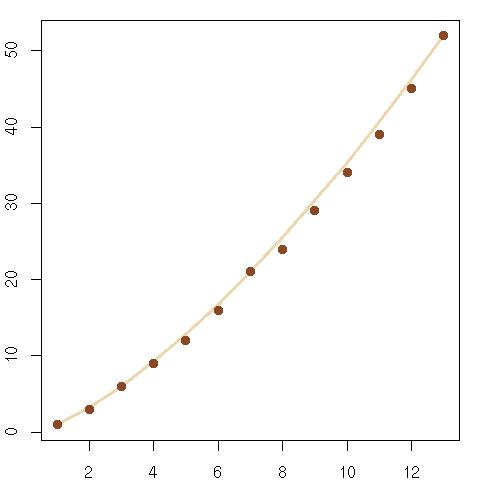Want to share your content on R-bloggers? click here if you have a blog, or here if you don't.

Given the wrong solution provided in Le Monde and comments from readers, I went to look a bit further on the Web for generic solutions to the rectangle problem. The most satisfactory version I have found so far is Mendelsohn’s in Mathematics Magazine, which gives as the maximal number$k^star = (n+1)(n^2+n+1)$

for a$Ntimes N=(n^2+n+1)times(n^2+n+1)$ grid. His theorem is based on the theory of projective planes and$n$ must be such that a projective plane of order$n$ exists, which seems equivalent to impose that$n$ is a prime number. The following graph plots the pairs$(N,k^star)$ when$N=1,ldots,13$ along with the known solutions, the fit being perfect for the values of$N$ of Mendelsohn’s form (i.e., 3, 7, 13).Unfortunately, the formula does not extend to other values of$N$, despite Menselsohn’s comment that using for$n$ the positive root of the equation$x^2+x+1=N$ and then replacing$n$ by nearby integers (in the maximal number) should work. (The first occurrence I found of a solution for a square-free set did not provide a generic solution, but only algorithmic directions. While it is restricted to squares. the link with fractal theory is nonetheless interesting.)

Filed under: Kids, R Tagged: fractal, Le Monde, mathematical puzzle, Mendelsohn, primes, projective planes